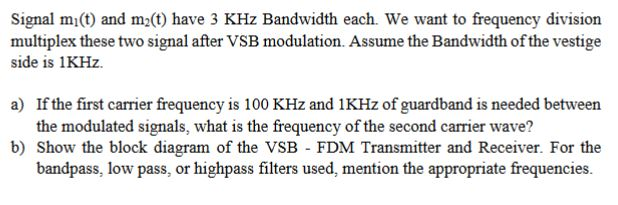Home / Answered Questions / Other / signal-mi-t-and-m-t-have-3-khz-bandwidth-each-we-want-to-frequency-division-multiplex-these-two-sign-aw258

# (Solved): Signal Mi(t) And M(t) Have 3 KHz Bandwidth Each. We Want To Frequency Division Multiplex These Two S...Signal mi(t) and m(t) have 3 KHz Bandwidth each. We want to frequency division multiplex these two signal after VSB modulation. Assume the Bandwidth of the vestige side is 1KHz. a) If the first carrier frequency is 100 KHz and 1KHz of guardband is needed between the modulated signals, what is the frequency of the second carrier wave? b) Show the block diagram of the VSB - FDM Transmitter and Receiver. For the bandpass, low pass, or highpass filters used, mention the appropriate frequencies.

We have an Answer from Expert